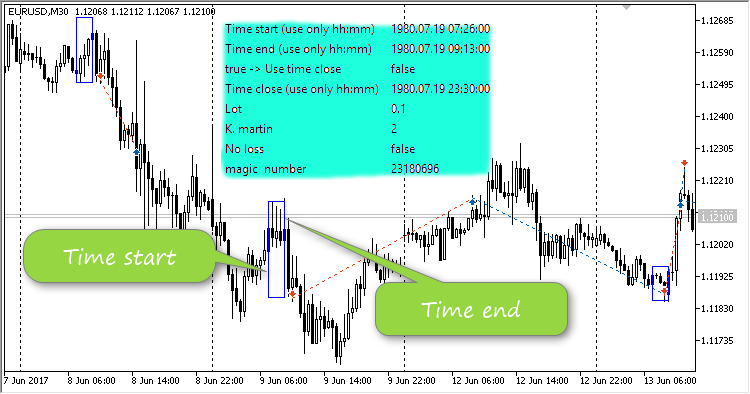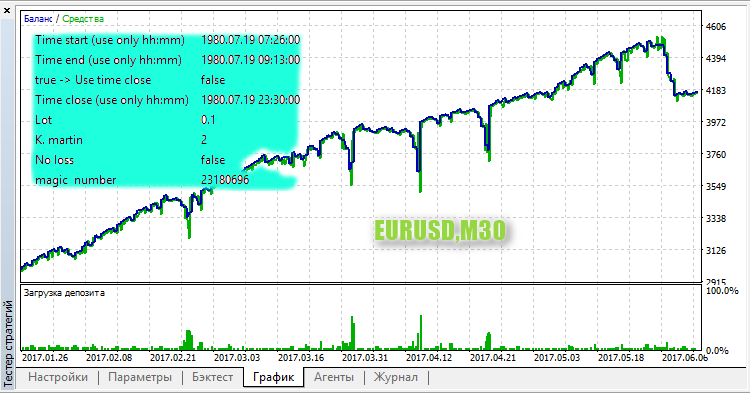# Nevalyashka_BreakdownLevel – expert for MetaTrader 5

• A+

Author of the idea — Vladimir Khlystov, author of the MQL5 code — barabashkakvn.

Trading strategy: the breakout of a High/Low in the selected time interval. To recover losing positions, the EA uses the "Nevalyashka" strategy and a martingale technique to increase the lot.

The EA calculates the High and Low price in the specified time interval from "Time start" to "Time end":If the price exceeds the High of this period, a BUY position is opened. If the price is below the Low, a SELL position is opened. StopLoss is set at an opposite border of the period, i.e. at a Low price for BUY positions and at a High price for SELL positions. TakeProfit is set based on the height of the control period.

If "Use time close" is set to true, the "Time close" parameter (time to close all positions) will be active.

Only hours and minutes are taken into account for parameters  "Time start""Time end" and "Time close".

Closure by StopLoss is monitored in OnTradeTransaction.

When "DEAL_ENTRY_OUT" (en: "Entry out") is found, check the comment field of this deal. If the comment contains "sl", it means the deal was closed by StopLoss:

```      if(deal_symbol==m_symbol.Name() && deal_magic==m_magic)
if(deal_entry==DEAL_ENTRY_OUT)
{
MqlDateTime str1;
TimeToStruct(TimeCurrent(),str1);
//--- there is a chance that this was a closure on the TakeProfit
if(StringFind(deal_comment,"tp",0)!=-1 || deal_profit>=0.0)
{
return;
}
//--- there is a chance that this was a closure on the StopLoss
if(StringFind(deal_comment,"sl",0)!=-1)
{
{
Print("A StopLoss closure has been detected!");

double loss=MathAbs(deal_profit/m_symbol.TickValue()/deal_volume);

if(deal_type==DEAL_TYPE_SELL) // the buy position is closed
{
double SL=m_symbol.Bid()+loss*m_symbol.Point();
double TP=m_symbol.Bid()-loss*m_symbol.Point();
double Lot=LotCheck(deal_volume*InpK_martin);
if(Lot==0.0)
return;
OpenSell(SL,TP,Lot,"Nevalyashka");

}
if(deal_type==DEAL_TYPE_BUY) // the sell position is closed
{
double Lot=LotCheck(deal_volume*InpK_martin);
if(Lot==0.0)
return;
}
return;
}
}
}
```

Open a position opposite to the closed one (if the deal type is "DEAL_TYPE_SELL", then a BUY position was closed; "DEAL_TYPE_BUY" means that a SELL position was closed), with an increased lot multiplied by K. martin. If a position was closed with a positive profit, the Expert Advisor waits for the start time of the next period "Time end", and repeats the above procedure.

"No loss" parameter means the breakeven; when half of position profit is reached, StopLoss is moved to the position open price.

Example if testing on EURUSD,M30: